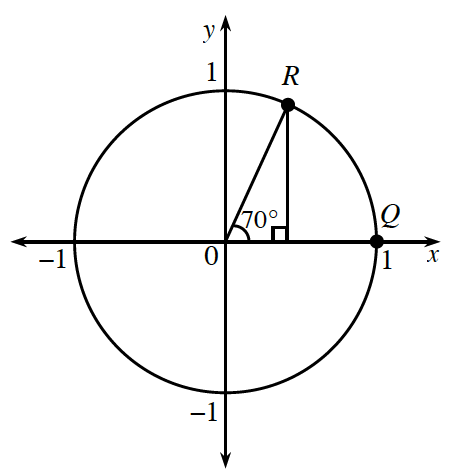### Home > INT3 > Chapter 9 > Lesson 9.1.4 > Problem9-54

9-54.

A $70^\circ$ angle is drawn for you in the unit circle at right.

1. Approximate the coordinates of point $R$.

$\left(0.3420, 0.9397\right)$

2. How could you represent the exact coordinates of point $R$?

$\left(\text{cos}\left(70^\circ\right), \text{sin}\left(70^\circ\right)\right)$

3. Show that the Pythagorean Identity works for this angle.

$\text{sin}^{2}\left(θ\right) + \text{cos}^{2}\left(θ\right) = 1$